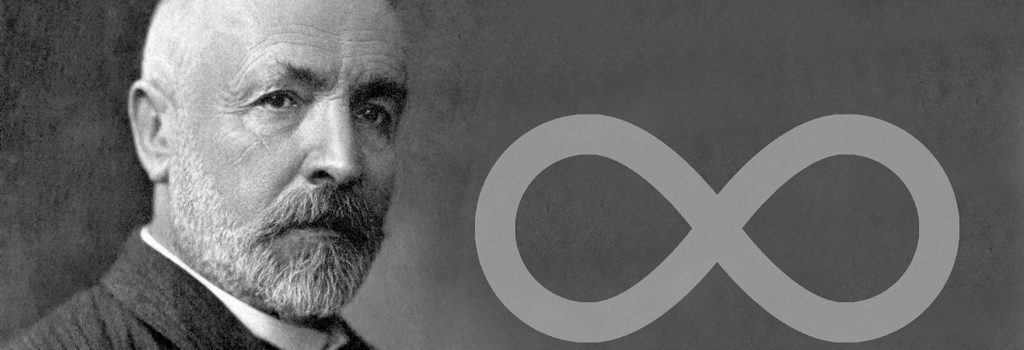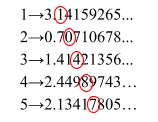## Does infinity exist?

Dhruvi Shukla. 05/29/2021Georg Cantor created many theorems relating to the mathematical infinity. (OpenMind BBVA)

Infinity. A value without limit. This concept created the law of continuity and allowed people to understand black holes, land rovers to mars, create new mathematical theorems and study the moon. Each discovery mentioned above comes from a different field in STEM. This is because there are three different types of infinities - the mathematical, the physical and the metaphysical. It is because of this that answering this question becomes more complicated. In this article, we’ll take an in-depth look into the mathematical infinities and see whether they exist or not.

During the late 19th century, the question of mathematical infinity arose in mathematician Georg Cantor’s mind, and he began investigating this idea. Through his extensive research, he discovered that there are many kinds of infinities in math, some larger than others. If all infinities were to be separated into groups, they would be countable infinities and uncountable infinities. He started by writing a sequence of numbers; let’s say, for example, that the even numbers are in one-to-one correspondence with another sequence within the real number system. By doing this, he found that the countable infinities are actually smaller than the uncountable infinities (also known as the continuum). This finding led to the continuum theory, which mathematicians are unable to disprove or solve even now. You may be asking how there could be infinities smaller than other infinities? So, to understand this, let’s take some whole numbers and even numbers in one-to-one correspondence; in other words, we are pairing up one whole number with an even. It should look something like this.

1→2 2→4 3→6 4→8 5→10

For simplicity’s sake, we will use numbers in chronological order. Cantor states that if we were to continue doing this infinitely, every whole number being paired up with an even number. This is strange to think about since there should be half as many even numbers as the whole, but when we are counting both numbers up to infinity, that stops being the case. Cantor continued using this method to research infinities and found various countable infinities until he started using irrational numbers. Even if he paired irrational numbers with another sequence of real numbers, he would still not be able to include every irrational number. In the exercise (above), it is safe to assume that if we went until infinity, we would be able to have all whole numbers paired with all even numbers. But this is not the case for irrational numbers. Repeating the exercise with irrational numbers will look something like this (remember this doesn’t need to be in chronological order):

1→3.14159265… 2→0.70710678… 3→1.41421356… 4→2.44989743… 5→2.13417805…

Now let’s imagine that we continue the pattern until infinity; we have all the whole and irrational values possible. Cantor states that there will still be irrational numbers which we will not find in our infinite list. To find this number for our list, circle all the numbers in your list in diagonals like so:Now write all these numbers down as a new irrational number like so: 0.10487… Now, add one to all these numbers like this: 0.21598… If we had an infinite list and used the method explained above for all the numbers in the list, we would have created a number that could not be found in the infinite list since one number from every number was changed. We see here what Cantor saw before concluding that there are infinities that are larger and smaller than one another; however, both infinities are still just ∞, and if we are to differentiate them, new axioms or equations that express the difference in these infinities must be created. It was Cantor’s theorem of the set theory, the continuum and the countable infinities that separated mathematical and physical infinities.

When news of these infinities came to Galileo Galileo and Kurt Gödel, they began playing around with Cantor’s Theorem themselves. Galileo later founded Galileo’s Paradox and Gödel later created Gödel’s incompleteness theorem. Galileo believed that having a less, greater or equal value only applies to number sets that are finite and not infinite. So using his paradox, we would state that “whether there are more irrational numbers than whole numbers, both infinite sets are neither greater, equal or less than each other”. However, Cantor believed this paradox was flawed and constructed a framework in which it is possible to compare countable infinities with one another and uncountable infinities with one another.

Infinities do exist, but in the mathematical system, there is not just one type of infinity but more than one with different sizes. However, it is important to remember that mathematical infinities are completely different from physical and metaphysical infinities.

Cover Photo: (OpenMind BBVA)Dhruvi Shukla